﻿ 船舶管理信息系统智能化发展探究
 舰船科学技术2020, Vol. 42Issue (6): 176-179    DOI: 10.3404/j.issn.1672-7649.2020.06.036PDF

1. 上海交通大学 船舶海洋与建筑工程学院，上海 200240;
2. 上海船舶研究设计院，上海 201203

Research on intelligent trend of ship management information system
LIU Dong1, YAN Wei-xiang2
1. School of Naval Architecture, Ocean and Civil Engineering, Shanghai Jiaotong University, Shanghai 201240, China;
2. Shanghai Merchant Ship Design and Research Institute, Shanghai 201203, China
Abstract: With the development of information technology, big data, artificial intelligence and other technologies, it’s the general trend that the ship management information system becomes more and more intelligent. Based on the present situation of ship management information system, this paper discusses the intelligent trend of ship-shore traffic management information system and ship management information system, and probes into the development trend of information sharing platform based on ship network, intelligent decision-making system, intelligent obstacle avoidance system and intelligent path planning or future ship management. A neural network model for intelligent decision-making and an autonomous obstacle avoidance and path planning model based on support vector machine are established. It provides a reference for the intelligent development of the ship management information system.
Key words: information sharing platform     intelligent obstacle avoidance system     intelligent path planning     neural network     support vector machine
0 引　言

1 船岸交通管理信息系统智能化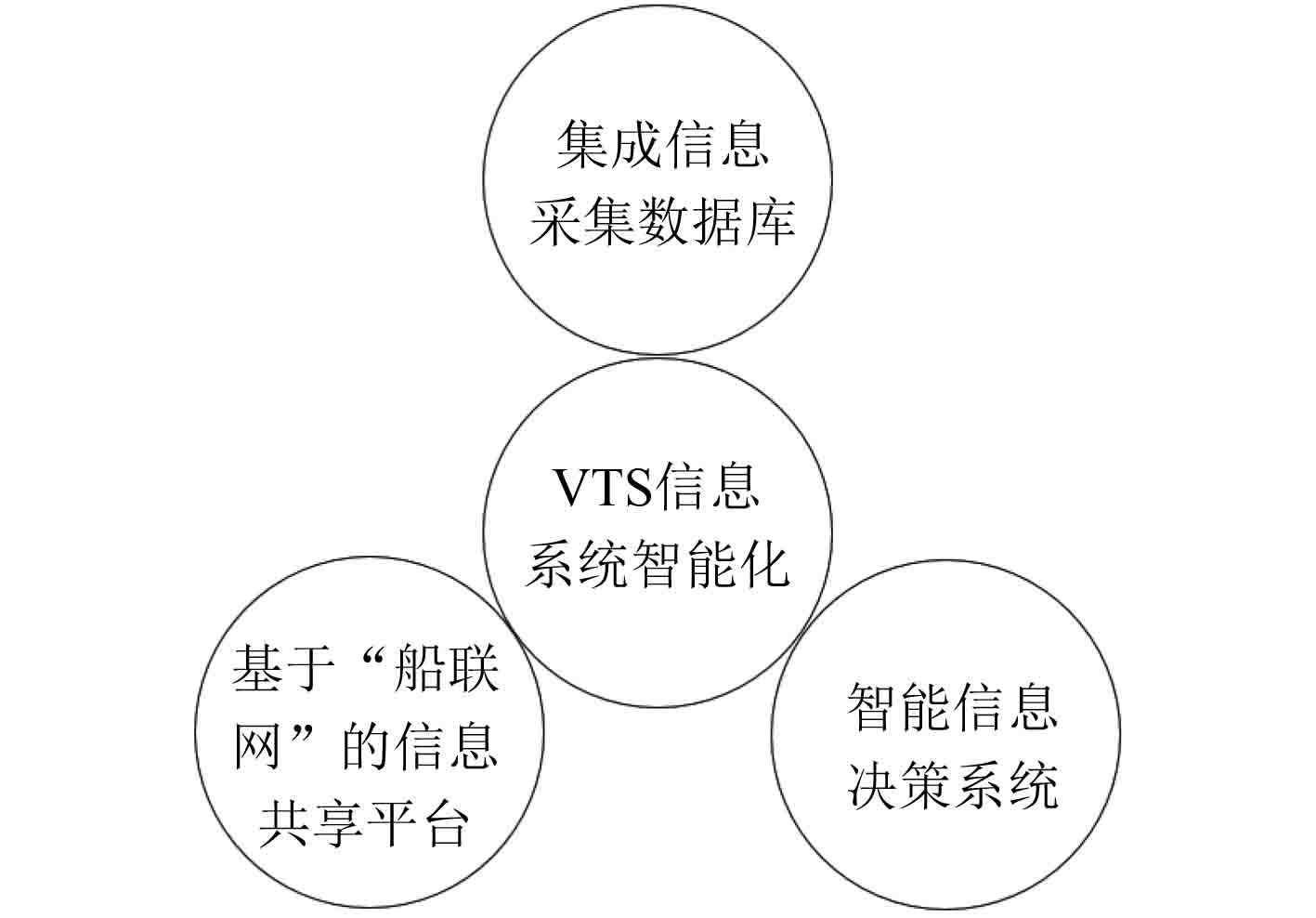图 1 VTS智能化体系结构 Fig. 1 Architecture of VTS intelligent system
1.1 基于“船联网”的信息共享平台

1.2 智能信息决策系统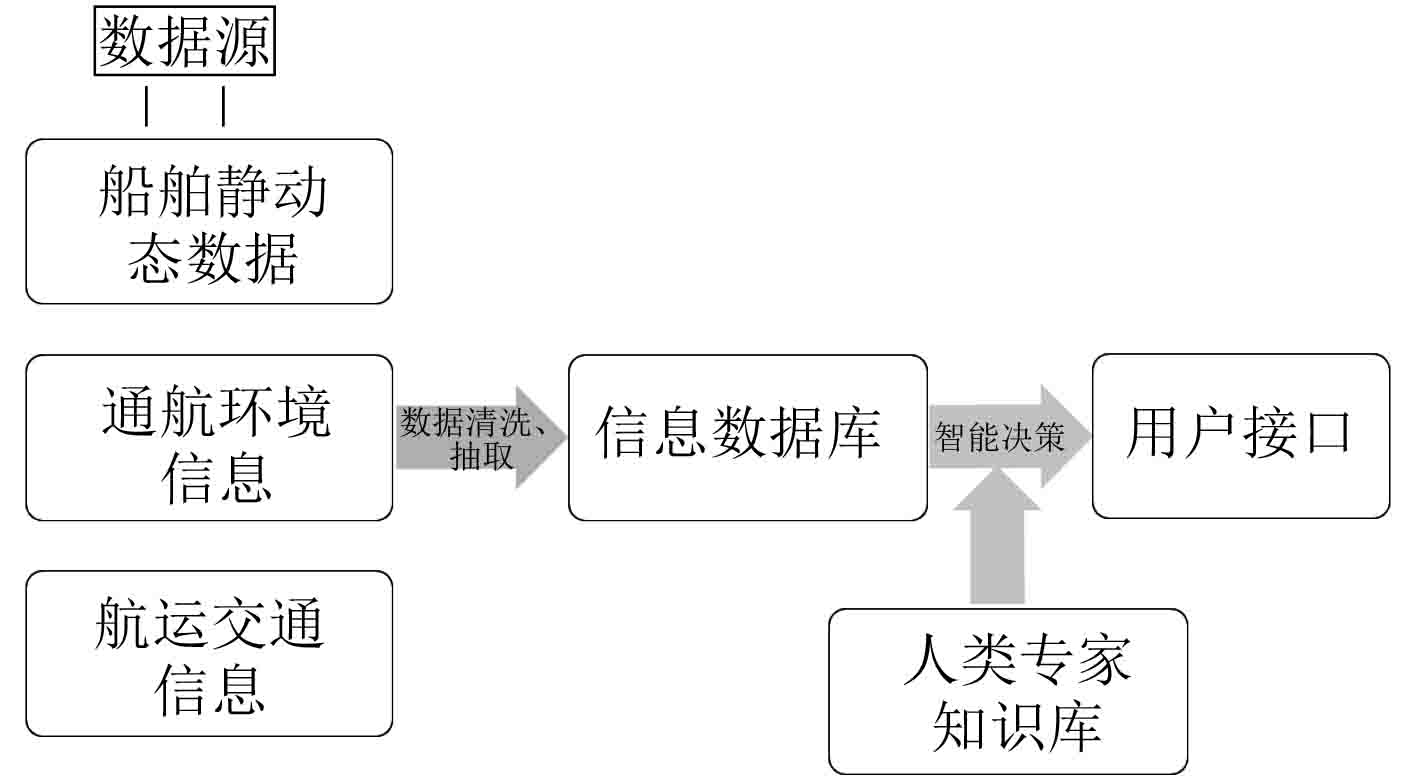图 2 智能决策分析信息流 Fig. 2 Information flow of intelligent decision-making system

 $\left\{ {\left( {{x^{(1)}},{s^{(1)}}} \right),\left( {{x^{(2)}},{s^{(2)}}} \right), \cdots ,\left( {{x^{(m)}},{s^{(m)}}} \right)} \right\}\text{。}$ (1)

 $\begin{split} {x^{(i)}} = & (x_1^{(i)},x_2^{(i)}, \cdots ,x_n^{(i)}) = \\ & ({a_1},{a_2}, \cdots ,{a_l},{b_1},{b_2}, \cdots ,{b_k},{c_1},{c_2}, \cdots ,{c_p}) \text{。} \end{split}$ (2)

s形函数（Sigmoid Function）作为激活函数，其表达公式为：

 $g(z) = \frac{1}{{1 + {e^{ - z}}}}\text{。}$ (3)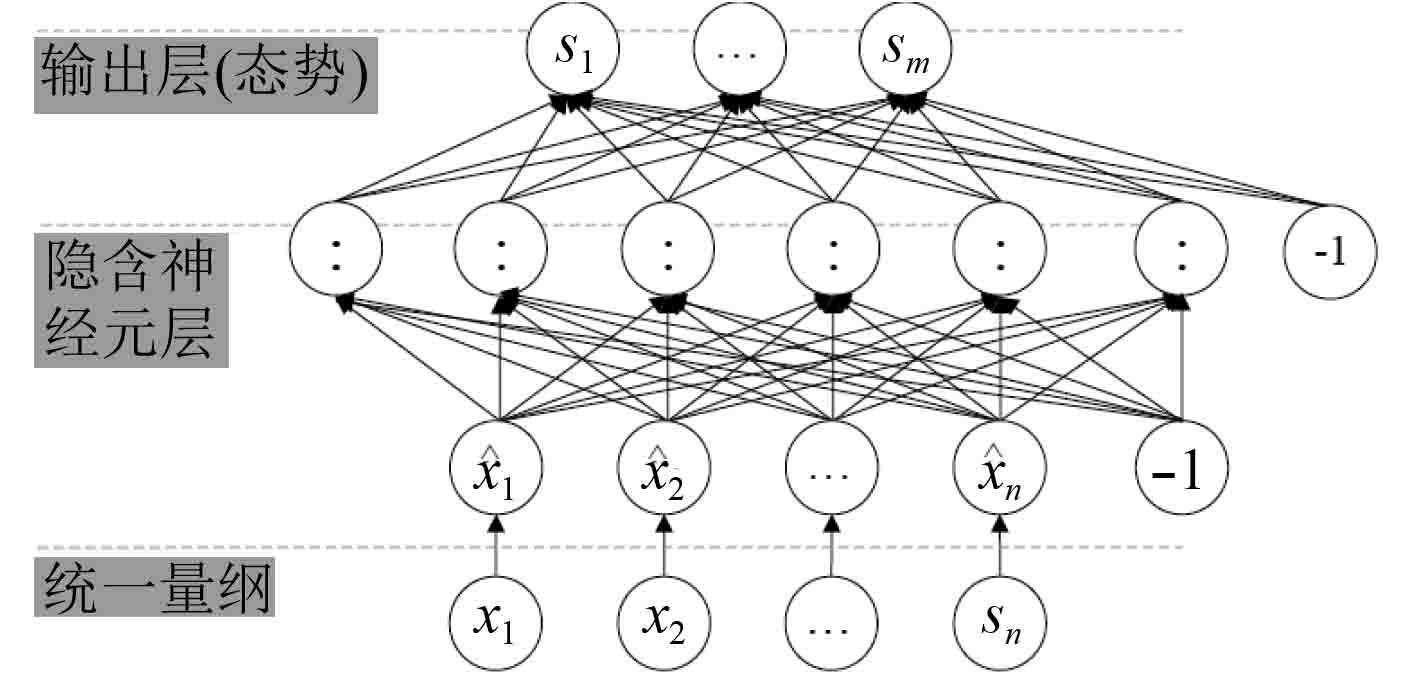图 3 神经网络结构模型 Fig. 3 Structural model of neural network

 ${\hat x_i} = g(\theta _{i0}^{(1)}{x_0} + \theta _{i1}^{(1)}{x_1} + \cdots ,\theta _{in}^{(1)}{x_n}) = g({\theta _i}x)\text{。}$ (4)

2 船舶管理信息系统智能化

2.1 自主避障系统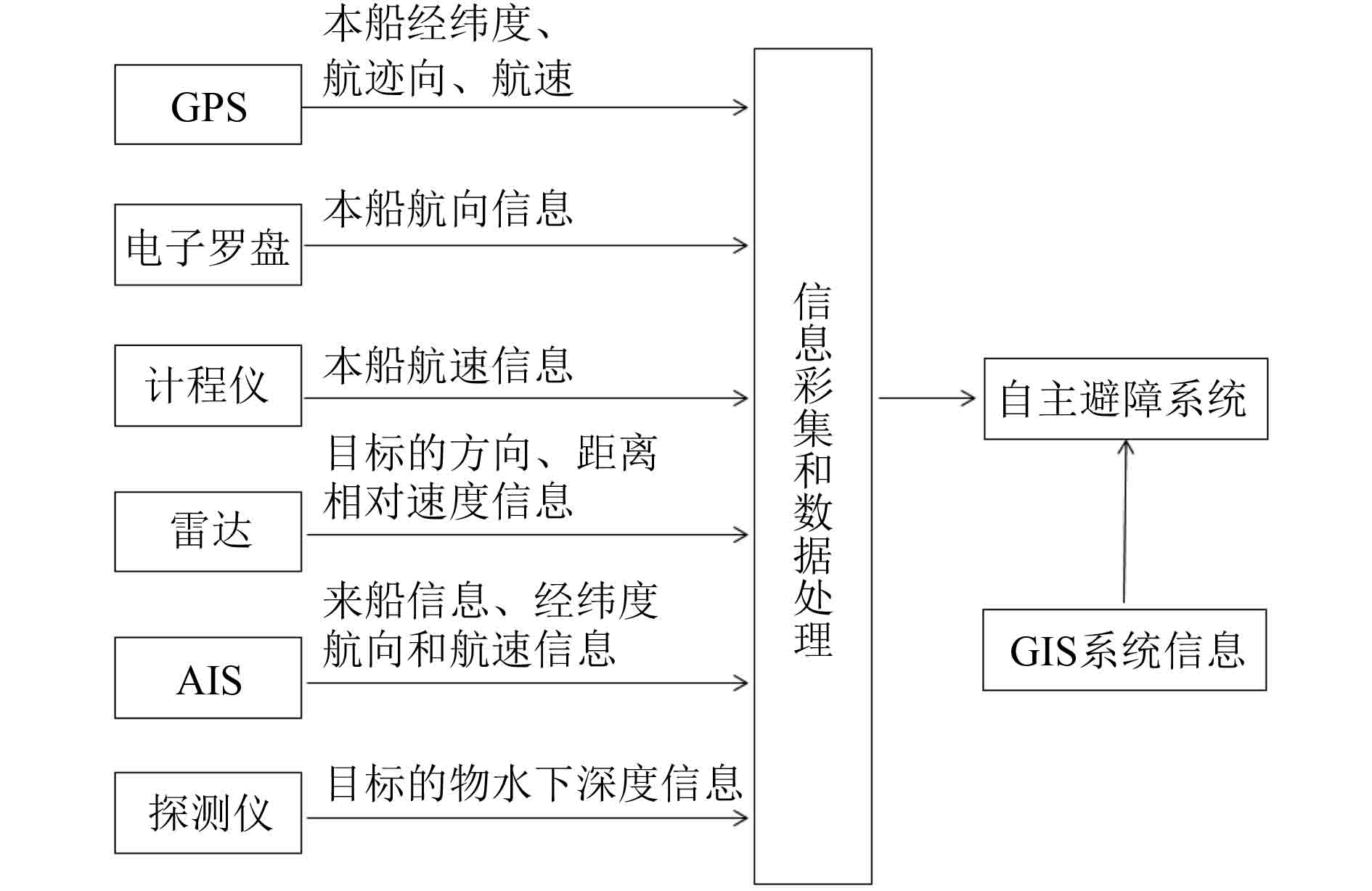图 4 自主避障信息系统结构 Fig. 4 Information structure of intelligent obstacle avoidance system
2.2 智能路径规划

2.3 基于支持向量机算法的自主避障和路径规划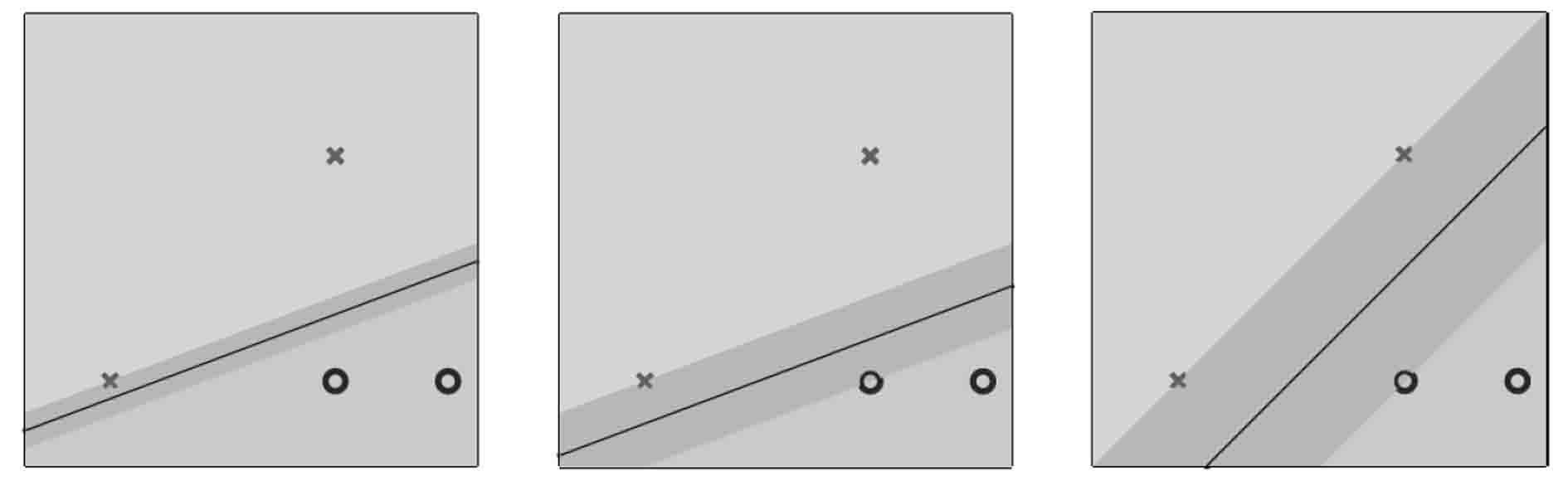图 5 支持向量机分类模型示例 Fig. 5 Example of support vector machine model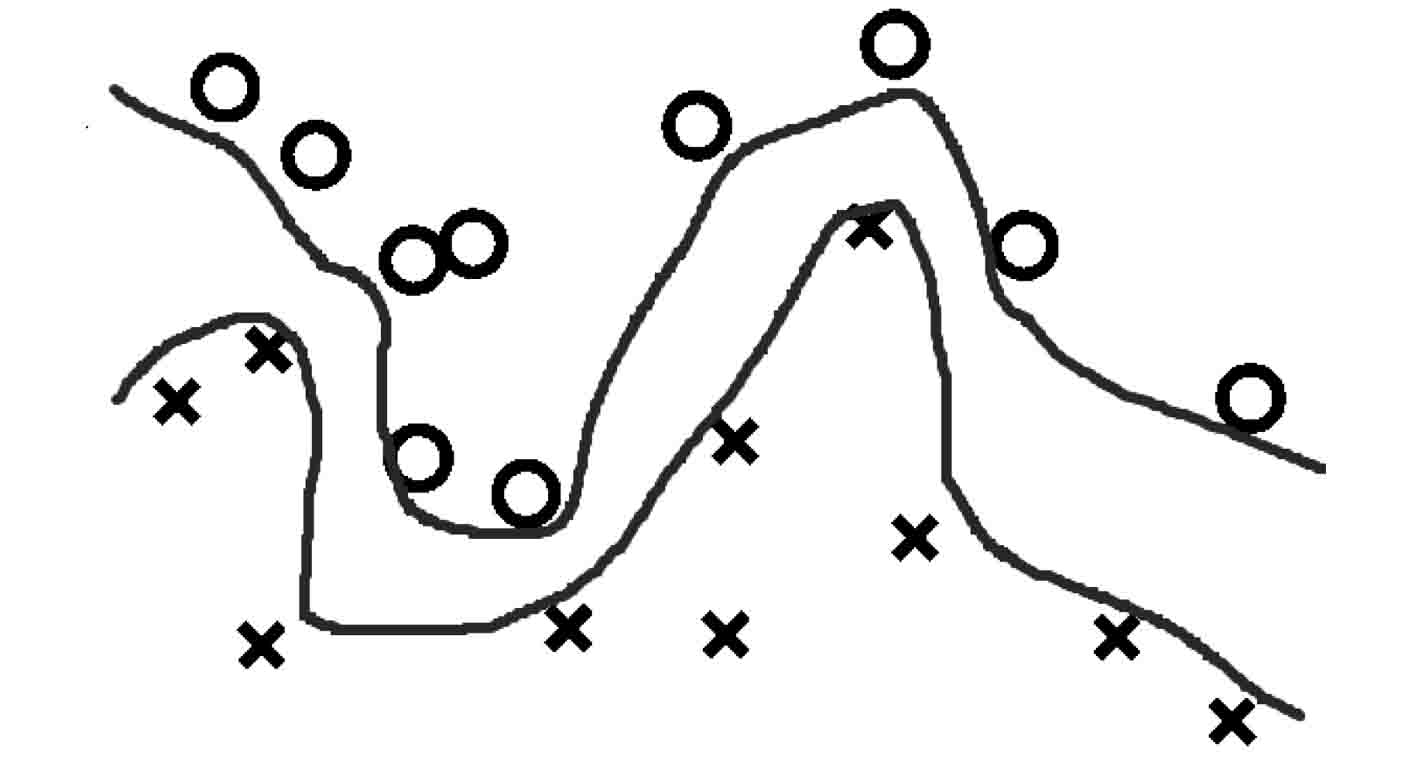图 6 某边界点和航线范围示例 Fig. 6 Example of boundary points and routes range

 $\begin{array}{l} \mathop {\max }\limits_{b,\overrightarrow \omega } \begin{array}{*{20}{c}} {}& \!\!\!\! {\mathop {\min }\limits_{n = 1,2, \cdots N,N + 1, \cdots M} }&{\displaystyle\frac{1}{{\left\| {\left. {\overrightarrow \omega } \right\|} \right.}}} \end{array}{y_n}({\overrightarrow \omega ^{\rm T}}\overrightarrow {{x_n}} \!+\! b)\text{，} \\ {\rm s.t.} \!\! \begin{array}{*{20}{c}} {}& \!\!\!\! {{y_n}({{\overrightarrow \omega }^{\rm T}}\overrightarrow {{x_n}} + b) > 0} \!\! &{n \!\!=\!\! 1,2, \cdots N,N \!+\! 1, \cdots M} \text{。} \end{array} \end{array}$ (5)

 $\begin{array}{l} \begin{array}{*{20}{c}} {}&{\mathop {\min }\limits_{\overrightarrow \alpha } }&{\displaystyle\frac{1}{2}} \end{array}{\overrightarrow \alpha ^T}{{ Q}_d}\overrightarrow \alpha - {\overrightarrow 1 ^T}\overrightarrow \alpha \text{，} \\ {\rm s.t.}\begin{array}{*{20}{c}} {}&{{{\overrightarrow y }^{\rm{T}}}\overrightarrow \alpha = 0;}&{} \end{array} \\ \begin{array}{*{20}{c}} {}&{}&{{\alpha _n}} \end{array} \geqslant 0,for\begin{array}{*{20}{c}} {n = 1,2, \cdots N,N + 1, \cdots M} \text{。} &{} \end{array} \\ \end{array}$ (6)

 ${{{K}}_Q}(\overrightarrow x ,\overrightarrow {{x'}} ) = {(\xi + \gamma {\overrightarrow x ^{\rm T}}\overrightarrow {{x'}} )^Q}\text{。}$ (7)

$\xi = 1$ $\gamma = 1$ Q=10，针对图6所示的边界点和航线范围可得到路径规划如图7所示。图 7 支持向量机模型所得的航线 Fig. 7 Routes derived from support vector machine

3 结　语

  郎咸周. 探讨船舶管理信息化现状及发展趋势[J]. 信息通信, 2014(9): 153. DOI:10.3969/j.issn.1673-1131.2014.09.109  ITU-R M. 1371. Technical characteristics for a universal shipborne automatic identification system using time division multiple access in the VHF maritime mobile band. 2001.  杨鑫. 智能船舶交通管理系统关键技术的研究与应用[D]. 大连: 大连海事大学, 2012.  熊和金, 陈德军. 智能信息处理[M]. 北京: 国防工业出版社, 2006.  魏伊, 薛彦卓. 船舶自动避碰仿真研究[J]. 大连海事大学学报, 2013, 39(2): 18-20, 24. DOI:10.3969/j.issn.1006-7736.2013.02.005  刘建业. 导航系统理论与应用[M]. 西安: 西北工业大学出版社, 2010.  陈佳. 无人驾驶救助船路径规划算法的研究[D]. 武汉: 武汉理工大学, 2013.  刘琨. 基于人工势场和蚁群算法的无人船路径规划研究[D]. 海口: 海南大学, 2016.